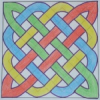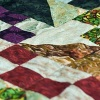# Resources tagged with: Factors and multiples

Filter by: Content type:
Age range:
Challenge level:

### There are 106 results

Broad Topics > Properties of Numbers > Factors and multiples### Cogs

##### Age 11 to 14Challenge Level

A and B are two interlocking cogwheels having p teeth and q teeth respectively. One tooth on B is painted red. Find the values of p and q for which the red tooth on B contacts every gap on the. . . .### Summing Consecutive Numbers

##### Age 11 to 14Challenge Level

15 = 7 + 8 and 10 = 1 + 2 + 3 + 4. Can you say which numbers can be expressed as the sum of two or more consecutive integers?### Got it for Two

##### Age 7 to 14Challenge Level

Got It game for an adult and child. How can you play so that you know you will always win?### Have You Got It?

##### Age 11 to 14Challenge Level

Can you explain the strategy for winning this game with any target?##### Age 11 to 14Challenge Level

List any 3 numbers. It is always possible to find a subset of adjacent numbers that add up to a multiple of 3. Can you explain why and prove it?### Beelines

##### Age 14 to 16Challenge Level

Is there a relationship between the coordinates of the endpoints of a line and the number of grid squares it crosses?### Hypotenuse Lattice Points

##### Age 14 to 16Challenge Level

The triangle OMN has vertices on the axes with whole number co-ordinates. How many points with whole number coordinates are there on the hypotenuse MN?### Shifting Times Tables

##### Age 11 to 14Challenge Level

Can you find a way to identify times tables after they have been shifted up or down?### Special Sums and Products

##### Age 11 to 14Challenge Level

Find some examples of pairs of numbers such that their sum is a factor of their product. eg. 4 + 12 = 16 and 4 × 12 = 48 and 16 is a factor of 48.### Got It

##### Age 7 to 14Challenge Level

A game for two people, or play online. Given a target number, say 23, and a range of numbers to choose from, say 1-4, players take it in turns to add to the running total to hit their target.### Diagonal Product Sudoku

##### Age 11 to 16Challenge Level

Given the products of diagonally opposite cells - can you complete this Sudoku?### DOTS Division

##### Age 14 to 16Challenge Level

Take any pair of two digit numbers x=ab and y=cd where, without loss of generality, ab > cd . Form two 4 digit numbers r=abcd and s=cdab and calculate: {r^2 - s^2} /{x^2 - y^2}.### Counting Factors

##### Age 11 to 14Challenge Level

Is there an efficient way to work out how many factors a large number has?### Three Times Seven

##### Age 11 to 14Challenge Level

A three digit number abc is always divisible by 7 when 2a+3b+c is divisible by 7. Why?### Hot Pursuit

##### Age 11 to 14Challenge Level

I added together the first 'n' positive integers and found that my answer was a 3 digit number in which all the digits were the same...##### Age 11 to 14Challenge Level

Great Granddad is very proud of his telegram from the Queen congratulating him on his hundredth birthday and he has friends who are even older than he is... When was he born?### Even So

##### Age 11 to 14Challenge Level

Find some triples of whole numbers a, b and c such that a^2 + b^2 + c^2 is a multiple of 4. Is it necessarily the case that a, b and c must all be even? If so, can you explain why?### Can You Find a Perfect Number?

##### Age 7 to 14### Take Three from Five

##### Age 14 to 16Challenge Level

Caroline and James pick sets of five numbers. Charlie chooses three of them that add together to make a multiple of three. Can they stop him?### Mathematical Swimmer

##### Age 11 to 14Challenge Level

Can you work out how many lengths I swim each day?### Counting Cogs

##### Age 7 to 14Challenge Level

Which pairs of cogs let the coloured tooth touch every tooth on the other cog? Which pairs do not let this happen? Why?### Drawing Celtic Knots

##### Age 11 to 14Challenge Level

Here is a chance to create some Celtic knots and explore the mathematics behind them.### What Numbers Can We Make?

##### Age 11 to 14Challenge Level

Imagine we have four bags containing a large number of 1s, 4s, 7s and 10s. What numbers can we make?### What Numbers Can We Make Now?

##### Age 11 to 14Challenge Level

Imagine we have four bags containing numbers from a sequence. What numbers can we make now?### Number Families

##### Age 11 to 14Challenge Level

How many different number families can you find?### Gabriel's Problem

##### Age 11 to 14Challenge Level

Gabriel multiplied together some numbers and then erased them. Can you figure out where each number was?### Cuboids

##### Age 11 to 14Challenge Level

Can you find a cuboid that has a surface area of exactly 100 square units. Is there more than one? Can you find them all?##### Age 7 to 14Challenge Level

I added together some of my neighbours' house numbers. Can you explain the patterns I noticed?### Repeaters

##### Age 11 to 14Challenge Level

Choose any 3 digits and make a 6 digit number by repeating the 3 digits in the same order (e.g. 594594). Explain why whatever digits you choose the number will always be divisible by 7, 11 and 13.### Star Product Sudoku

##### Age 11 to 16Challenge Level

The puzzle can be solved by finding the values of the unknown digits (all indicated by asterisks) in the squares of the $9\times9$ grid.### Inclusion Exclusion

##### Age 11 to 14Challenge Level

How many integers between 1 and 1200 are NOT multiples of any of the numbers 2, 3 or 5?### Factor Lines

##### Age 7 to 14Challenge Level

Arrange the four number cards on the grid, according to the rules, to make a diagonal, vertical or horizontal line.### Funny Factorisation

##### Age 11 to 16Challenge Level

Using the digits 1 to 9, the number 4396 can be written as the product of two numbers. Can you find the factors?### Factor Track

##### Age 7 to 14Challenge Level

Factor track is not a race but a game of skill. The idea is to go round the track in as few moves as possible, keeping to the rules.### Big Powers

##### Age 11 to 16Challenge Level

Three people chose this as a favourite problem. It is the sort of problem that needs thinking time - but once the connection is made it gives access to many similar ideas.### How Old Are the Children?

##### Age 11 to 14Challenge Level

A student in a maths class was trying to get some information from her teacher. She was given some clues and then the teacher ended by saying, "Well, how old are they?"### Two Much

##### Age 11 to 14Challenge Level

Explain why the arithmetic sequence 1, 14, 27, 40, ... contains many terms of the form 222...2 where only the digit 2 appears.### Charlie's Delightful Machine

##### Age 11 to 16Challenge Level

Here is a machine with four coloured lights. Can you develop a strategy to work out the rules controlling each light?### Factors and Multiples Game

##### Age 7 to 16Challenge Level

A game in which players take it in turns to choose a number. Can you block your opponent?### Exploring Simple Mappings

##### Age 11 to 14Challenge Level

Explore the relationship between simple linear functions and their graphs.### Transposition Cipher

##### Age 11 to 16Challenge Level

Can you work out what size grid you need to read our secret message?### Power Crazy

##### Age 11 to 14Challenge Level

What can you say about the values of n that make $7^n + 3^n$ a multiple of 10? Are there other pairs of integers between 1 and 10 which have similar properties?### What a Joke

##### Age 14 to 16Challenge Level

Each letter represents a different positive digit AHHAAH / JOKE = HA What are the values of each of the letters?### Dozens

##### Age 7 to 14Challenge Level

Do you know a quick way to check if a number is a multiple of two? How about three, four or six?### Stars

##### Age 11 to 14Challenge Level

Can you find a relationship between the number of dots on the circle and the number of steps that will ensure that all points are hit?### Alison's Quilt

##### Age 11 to 14Challenge Level

Nine squares are fitted together to form a rectangle. Can you find its dimensions?### Why 24?

##### Age 14 to 16Challenge Level

Take any prime number greater than 3 , square it and subtract one. Working on the building blocks will help you to explain what is special about your results.### Different by One

##### Age 14 to 16Challenge Level

Can you make lines of Cuisenaire rods that differ by 1?### Data Chunks

##### Age 14 to 16Challenge Level

Data is sent in chunks of two different sizes - a yellow chunk has 5 characters and a blue chunk has 9 characters. A data slot of size 31 cannot be exactly filled with a combination of yellow and. . . .### LCM Sudoku II

##### Age 11 to 18Challenge Level

You are given the Lowest Common Multiples of sets of digits. Find the digits and then solve the Sudoku.